# igus® terms in alphabetical order

Application icons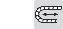Unsupported - short travels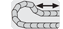Gliding - long travels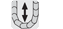Vertical hanging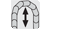Vertical standing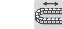Side-mounted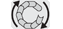Rotary motion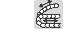Horizontal + vertical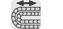Nested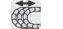Side by side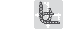Combined motions

Short cuts and formulas

 Short cut Describtion α = Rotation angle [°] ΔM = Deviation of the center point [inch] a = Acceleration [ft/s 2] AR = Outer radius, energy chain [inch] Ba = Outer energy chain width [inch] Bi = Inner energy chain width [inch] BRa = Guide trough outer width [inch] BRi = Guide trough inner width [inch] D = Over length energy chain radius in final position [inch] D2 = Over length for long travels gliding [inch] FL B = Unsupported length with sag [ft] FL G = Unsupported straight length [ft] FL U = Unsupported lower run [ft] FZmax = Maximum additional load [lbs/ft] H = Nominal clearence height [inch] ha = Outer energy chainheight [inch] HF = Required clearance height [inch] hi = Inner height of energy chain [inch] HRa = Outer height of guide trough [inch] HRi = Inner height of guide trough [inch] IR = Inner radius of energy chain [inch] K = Add-on for bending radius [inch] K2 = Further add-on if the mounting bracket location is set lower (for long travels) [inch] LK = energy chain length [inch] n = Number of links  nMon = Number of installation sets (left/right)  nRi = Number of trough-sets (left/right)  R = Bending radius [inch] RBR = Reverse bending radius [inch] S = Length of travel [inch] S/2 = Half length of travel [inch] T = Pitch [inch] v = Speed (travel) [ft/s] X2 = Inner machine-construction space (twisterchain) [inch] X2 = Outer radius chain, including clearance [inch]

 Formula Describtion SFLB = 2 * FL B = Calculation of maximum travel length, unsupported with sag SFLG = 2 * FL G = Calculation of maximum travel length, unsupported straight BRI ≥ Ba + 5 = Calculation of the minimum guide trough width HRI ≥ 2 * ha = Calculation of the minimum guide trough height K = π * R = Add-on for bending radius LK = S/2 + ΔM + K = Calculation of chain lengths - for all types of applications, fixed end is outside the center of travel (with FLG, FLB and ΔM) LK = S/2 + K = Calculation of chain lengths for all types of applications, fixed end in the center of travel,except rotary movements and most long travels (with FLG and FLB) [m] LK = S/2 + K 2 = Calculation of chain lengths for long travels, fixed end in the center of travel

Links